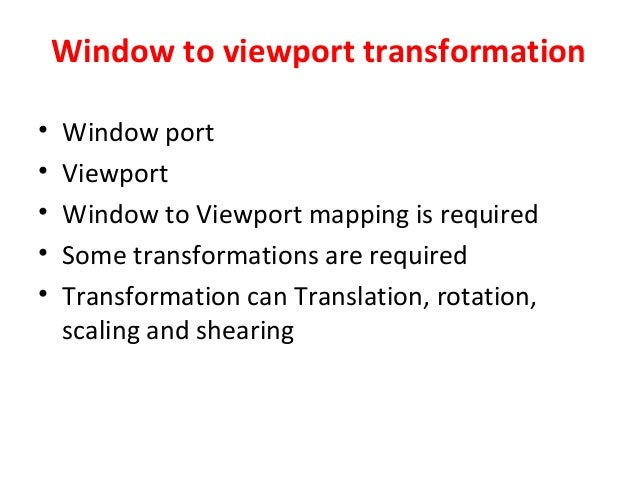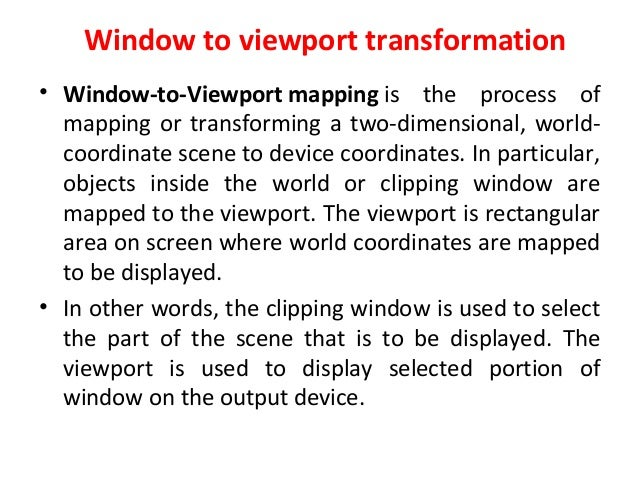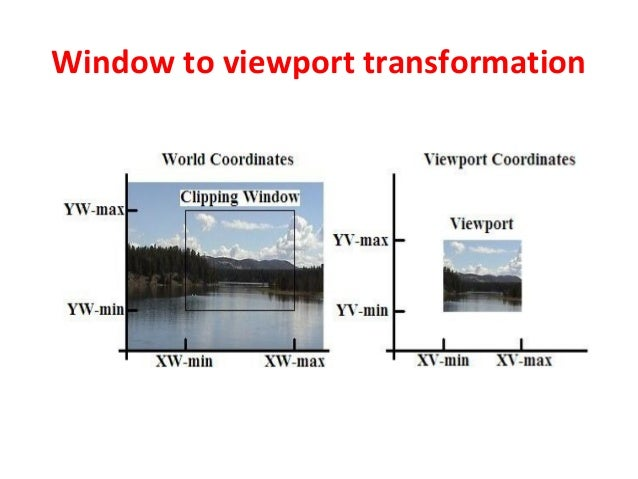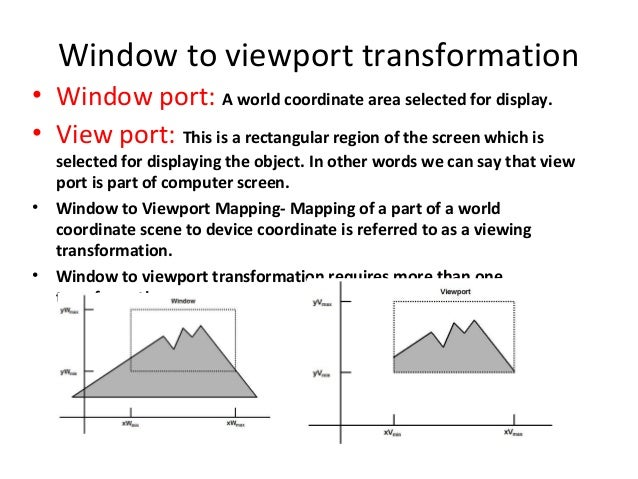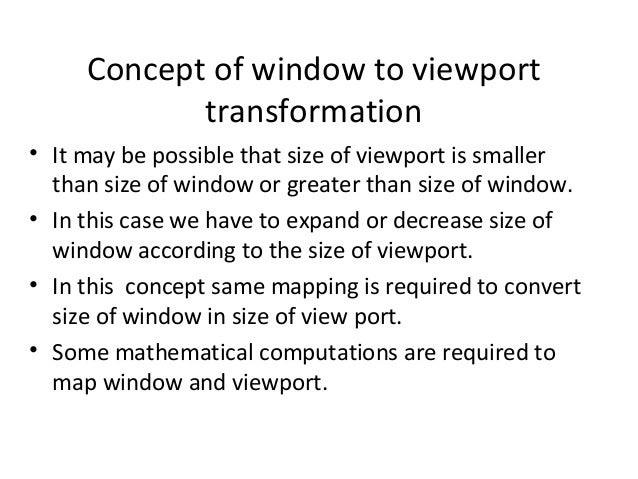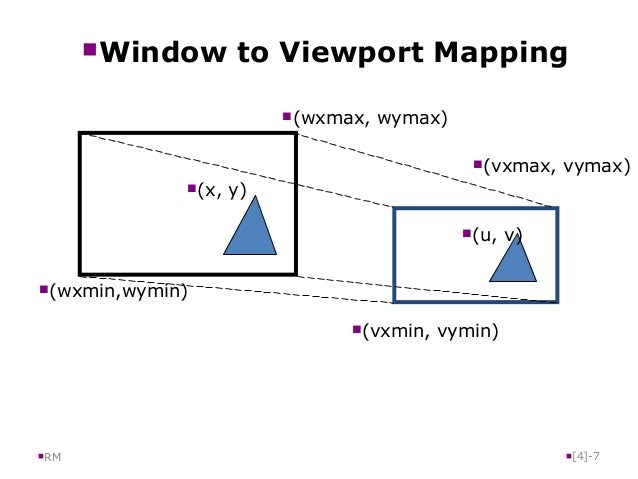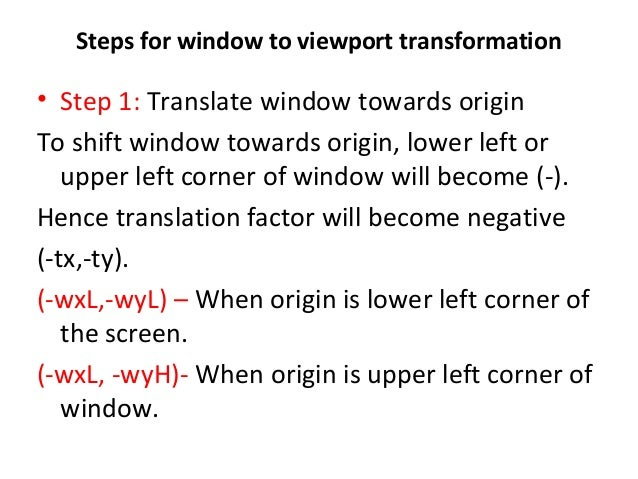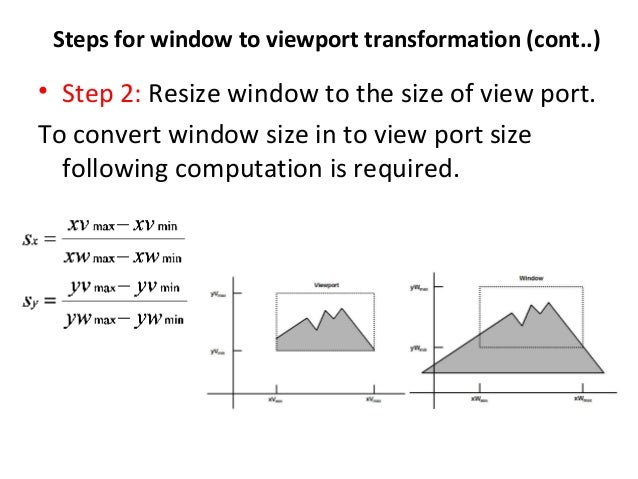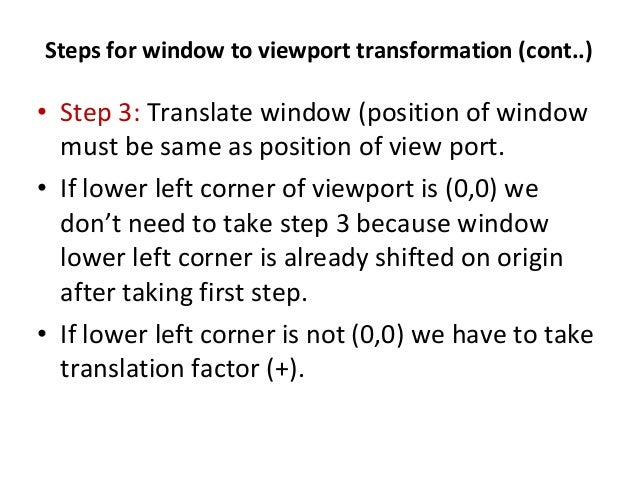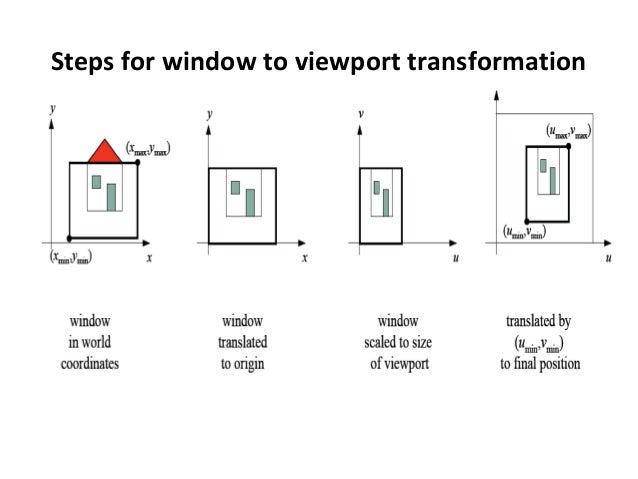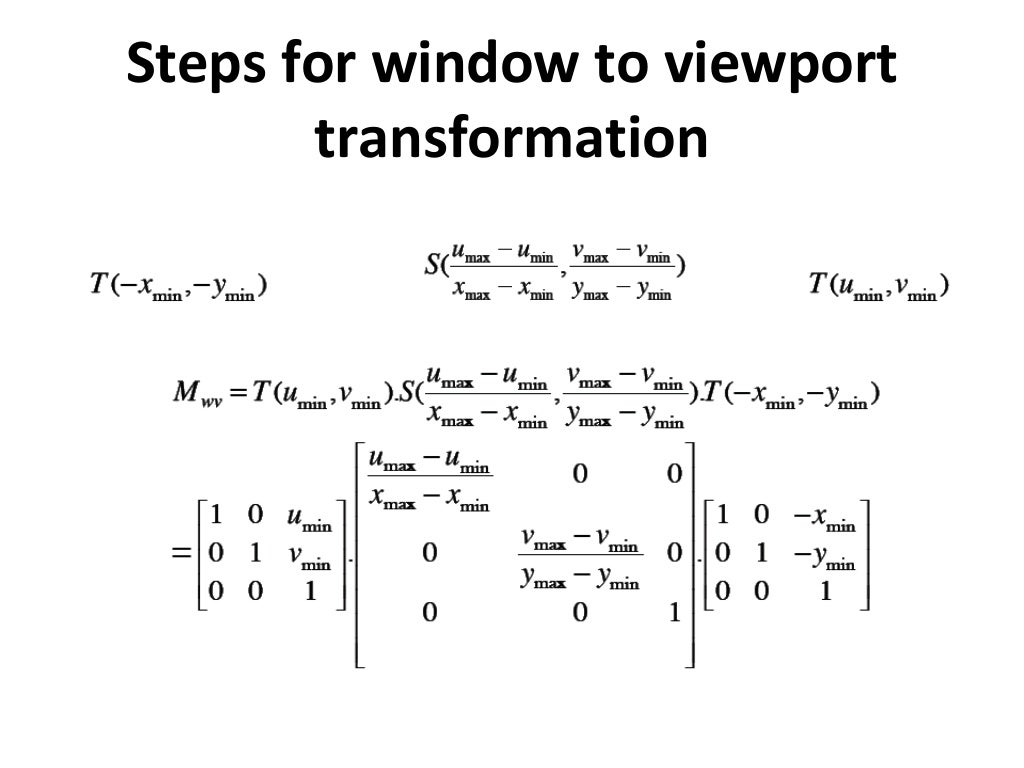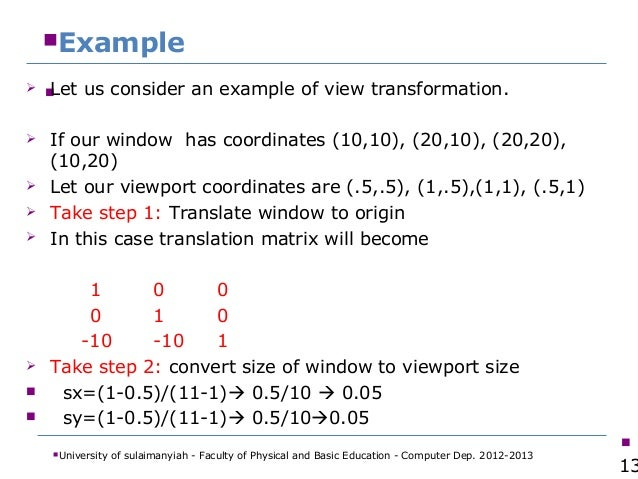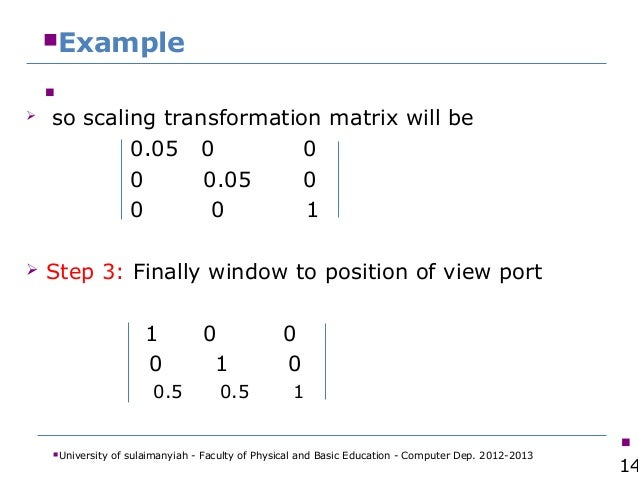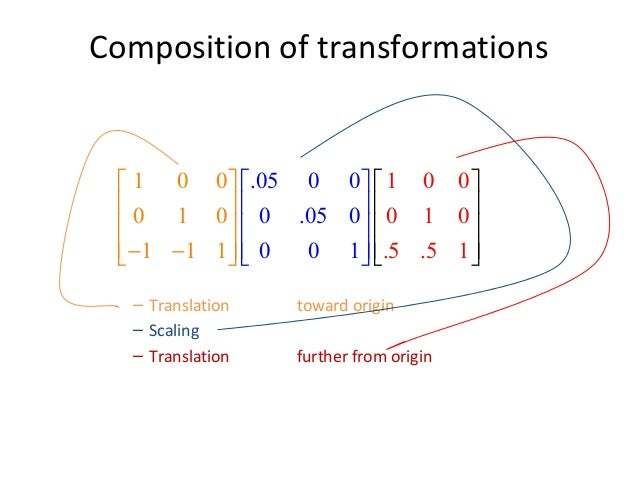Posted in Computer graphics MCA

## Parallel Projection

Parallel projection discards z-coordinate and parallel lines from each vertex on the object are extended until they intersect the view plane. In parallel projection, we specify a direction of projection instead of center of projection.

In parallel projection, the distance from the center of projection to project plane is infinite. In this type of projection, we connect the projected vertices by line segments which correspond to connections on the original object.

Parallel projections are less realistic, but they are good for exact measurements. In this type of projections, parallel lines remain parallel and angles are not preserved. Various types of parallel projections are shown in the following hierarchy.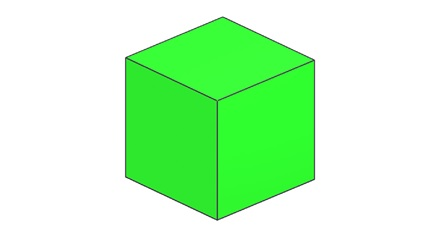## Perspective Projection

In perspective projection, the distance from the center of projection to project plane is finite and the size of the object varies inversely with distance which looks more realistic.

The distance and angles are not preserved and parallel lines do not remain parallel. Instead, they all converge at a single point called center of projection or projection reference point. There are 3 types of perspective projections which are shown in the following chart.

• One point perspective projection is simple to draw.
• Two point perspective projection gives better impression of depth.
• Three point perspective projection is most difficult to draw.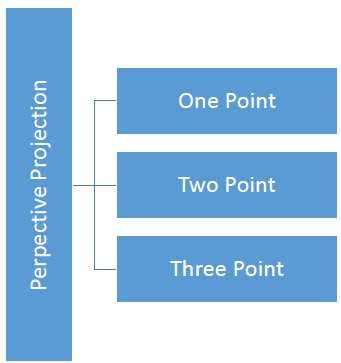The following figure shows all the three types of perspective projection −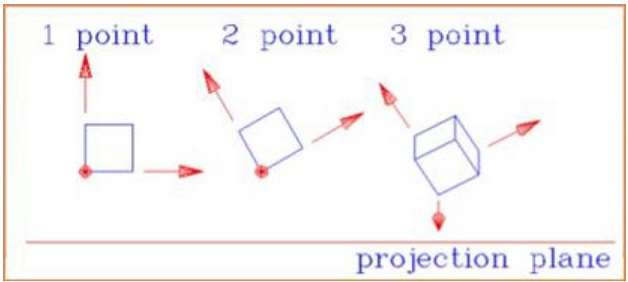Posted in Computer graphics MCA

## Rotation

3D rotation is not same as 2D rotation. In 3D rotation, we have to specify the angle of rotation along with the axis of rotation. We can perform 3D rotation about X, Y, and Z axes. They are represented in the matrix form as below −

Rx(θ)=⎡⎣⎢⎢⎢10000cosθsinθ00sinθcosθ00001⎤⎦⎥⎥⎥Ry(θ)=⎡⎣⎢⎢⎢cosθ0sinθ00100sinθ0cosθ00001⎤⎦⎥⎥⎥Rz(θ)=⎡⎣⎢⎢⎢cosθsinθ00sinθcosθ0000100001⎤⎦⎥⎥⎥Rx(θ)=[10000cosθ−sinθ00sinθcosθ00001]Ry(θ)=[cosθ0sinθ00100−sinθ0cosθ00001]Rz(θ)=[cosθ−sinθ00sinθcosθ0000100001]

The following figure explains the rotation about various axes −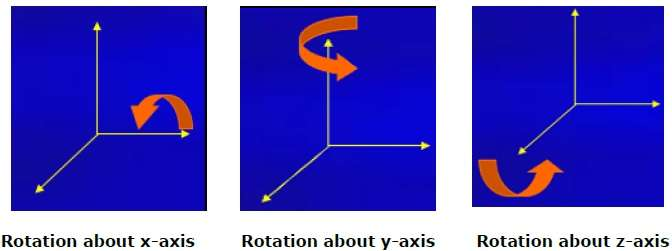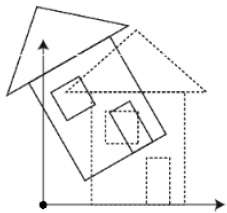## Scaling

You can change the size of an object using scaling transformation. In the scaling process, you either expand or compress the dimensions of the object. Scaling can be achieved by multiplying the original coordinates of the object with the scaling factor to get the desired result. The following figure shows the effect of 3D scaling −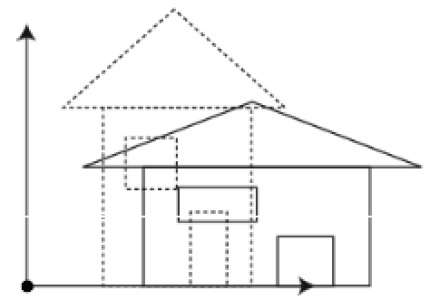In 3D scaling operation, three coordinates are used. Let us assume that the original coordinates are (X, Y, Z), scaling factors are (SX,SY,Sz)(SX,SY,Sz) respectively, and the produced coordinates are (X’, Y’, Z’). This can be mathematically represented as shown below −

S=⎡⎣⎢⎢⎢⎢Sx0000Sy0000Sz00001⎤⎦⎥⎥⎥⎥S=[Sx0000Sy0000Sz00001]

P’ = P∙S

[XYZ1]=[XYZ1]⎡⎣⎢⎢⎢⎢Sx0000Sy0000Sz00001⎤⎦⎥⎥⎥⎥[X′Y′Z′1]=[XYZ1][Sx0000Sy0000Sz00001]

=[X.SxY.SyZ.Sz1]=[X.SxY.SyZ.Sz1]

## Shear

A transformation that slants the shape of an object is called the shear transformation. Like in 2D shear, we can shear an object along the X-axis, Y-axis, or Z-axis in 3D.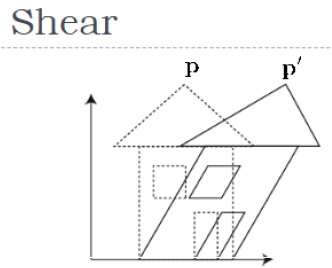As shown in the above figure, there is a coordinate P. You can shear it to get a new coordinate P’, which can be represented in 3D matrix form as below −

Sh=⎡⎣⎢⎢⎢⎢1shxyshxz0shyx1shyz0shzxshzy100001⎤⎦⎥⎥⎥⎥Sh=[1shxyshxz0shyx1shyz0shzxshzy100001]

P’ = P ∙ Sh

X=X+ShyxY+ShzxZX′=X+ShxyY+ShxzZ

Y=ShxyX+Y+shzyZY′=ShyxX+Y+shyzZ

Z=ShxzX+ShyzY+ZZ′=ShzxX+ShzyY+Z

## Transformation Matrices

Transformation matrix is a basic tool for transformation. A matrix with n x m dimensions is multiplied with the coordinate of objects. Usually 3 x 3 or 4 x 4 matrices are used for transformation. For example, consider the following matrix for various operation.

 T=⎡⎣⎢⎢⎢⎢100tx010ty001tz0001⎤⎦⎥⎥⎥⎥T=[100001000010txtytz1] S=⎡⎣⎢⎢⎢⎢Sx0000Sy0000Sz00001⎤⎦⎥⎥⎥⎥S=[Sx0000Sy0000Sz00001] Sh=⎡⎣⎢⎢⎢⎢1shxyshxz0shyx1shyz0shzxshzy100001⎤⎦⎥⎥⎥⎥Sh=[1shxyshxz0shyx1shyz0shzxshzy100001] Translation Matrix Scaling Matrix Shear Matrix Rx(θ)=⎡⎣⎢⎢⎢10000cosθsinθ00−sinθcosθ00001⎤⎦⎥⎥⎥Rx(θ)=[10000cosθ−sinθ00sinθcosθ00001] Ry(θ)=⎡⎣⎢⎢⎢cosθ0−sinθ00100sinθ0cosθ00001⎤⎦⎥⎥⎥Ry(θ)=[cosθ0sinθ00100−sinθ0cosθ00001] Rz(θ)=⎡⎣⎢⎢⎢cosθsinθ00−sinθcosθ0000100001⎤⎦⎥⎥⎥Rz(θ)=[cosθ−sinθ00sinθcosθ0000100001] Rotation Matrix
Posted in Computer graphics MCA

### 3-Dimensional Display Methods – 3D Computer Graphics

In the 2D system, we use only two coordinates X and Y but in 3D, an extra coordinate Z is added. 3D graphics techniques and their application are fundamental to the entertainment, games, and computer-aided design industries. It is a continuing area of research in scientific visualization.

Furthermore, 3D graphics components are now a part of almost every personal computer and, although traditionally intended for graphics-intensive software such as games, they are increasingly being used by other applications.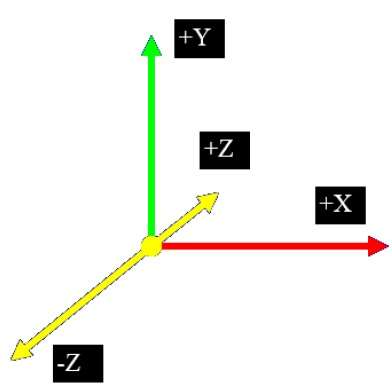## Parallel Projection

Parallel projection discards z-coordinate and parallel lines from each vertex on the object are extended until they intersect the view plane. In parallel projection, we specify a direction of projection instead of center of projection.

In parallel projection, the distance from the center of projection to project plane is infinite. In this type of projection, we connect the projected vertices by line segments which correspond to connections on the original object.

Parallel projections are less realistic, but they are good for exact measurements. In this type of projections, parallel lines remain parallel and angles are not preserved. Various types of parallel projections are shown in the following hierarchy.## Orthographic Projection

In orthographic projection the direction of projection is normal to the projection of the plane. There are three types of orthographic projections −

• Front Projection
• Top Projection
• Side Projection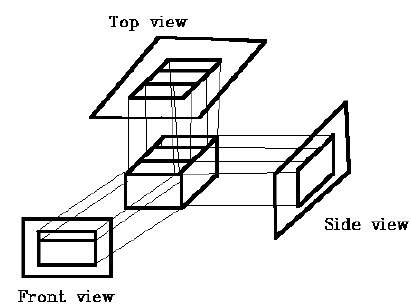## Oblique Projection

In oblique projection, the direction of projection is not normal to the projection of plane. In oblique projection, we can view the object better than orthographic projection.

There are two types of oblique projections − Cavalier and Cabinet. The Cavalier projection makes 45° angle with the projection plane. The projection of a line perpendicular to the view plane has the same length as the line itself in Cavalier projection. In a cavalier projection, the foreshortening factors for all three principal directions are equal.

The Cabinet projection makes 63.4° angle with the projection plane. In Cabinet projection, lines perpendicular to the viewing surface are projected at ½ their actual length. Both the projections are shown in the following figure −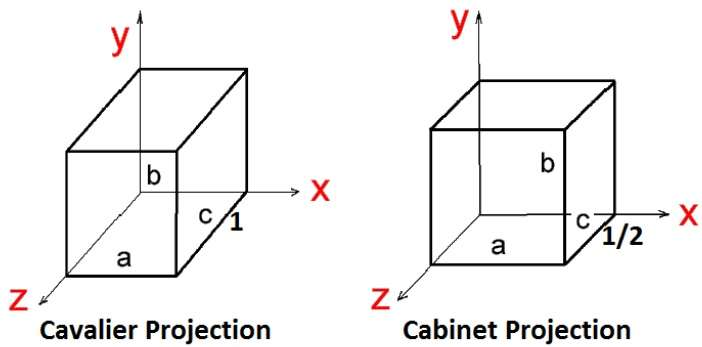## Isometric Projections

Orthographic projections that show more than one side of an object are called axonometric orthographic projections. The most common axonometric projection is an isometric projection where the projection plane intersects each coordinate axis in the model coordinate system at an equal distance. In this projection parallelism of lines are preserved but angles are not preserved. The following figure shows isometric projection −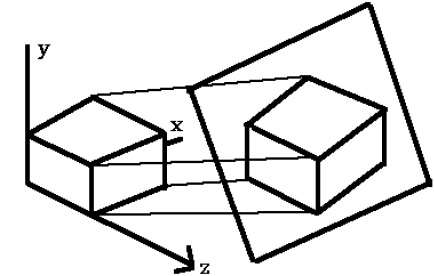## Perspective Projection

In perspective projection, the distance from the center of projection to project plane is finite and the size of the object varies inversely with distance which looks more realistic.

The distance and angles are not preserved and parallel lines do not remain parallel. Instead, they all converge at a single point called center of projection or projection reference point. There are 3 types of perspective projections which are shown in the following chart.

• One point perspective projection is simple to draw.
• Two point perspective projection gives better impression of depth.
• Three point perspective projection is most difficult to draw.The following figure shows all the three types of perspective projection −## Translation

In 3D translation, we transfer the Z coordinate along with the X and Y coordinates. The process for translation in 3D is similar to 2D translation. A translation moves an object into a different position on the screen.

The following figure shows the effect of translation −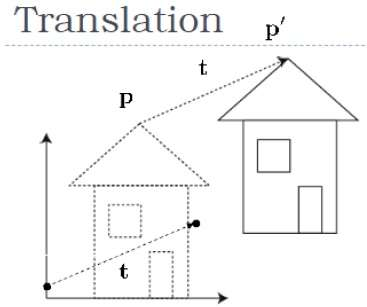A point can be translated in 3D by adding translation coordinate (tx,ty,tz)(tx,ty,tz) to the original coordinate (X, Y, Z) to get the new coordinate (X’, Y’, Z’).

T=⎡⎣⎢⎢⎢⎢100tx010ty001tz0001⎤⎦⎥⎥⎥⎥T=[100001000010txtytz1]

P’ = P∙T

[XYZ1]=[XYZ1]⎡⎣⎢⎢⎢⎢100tx010ty001tz0001⎤⎦⎥⎥⎥⎥[X′Y′Z′1]=[XYZ1][100001000010txtytz1]

=[X+txY+tyZ+tz1]

Posted in Computer graphics MCA

### Exterior clipping in computer graphics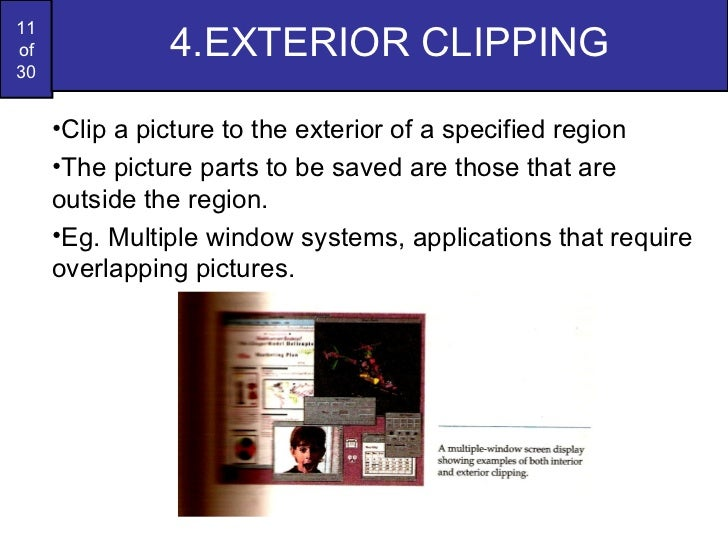Posted in Computer graphics MCA

## Text Clipping

Various techniques are used to provide text clipping in a computer graphics. It depends on the methods used to generate characters and the requirements of a particular application. There are three methods for text clipping which are listed below −

• All or none string clipping
• All or none character clipping
• Text clipping

The following figure shows all or none string clipping −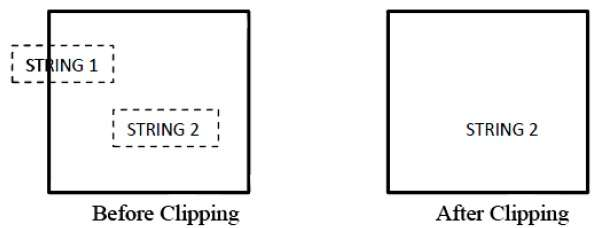In all or none string clipping method, either we keep the entire string or we reject entire string based on the clipping window. As shown in the above figure, STRING2 is entirely inside the clipping window so we keep it and STRING1 being only partially inside the window, we reject.

The following figure shows all or none character clipping −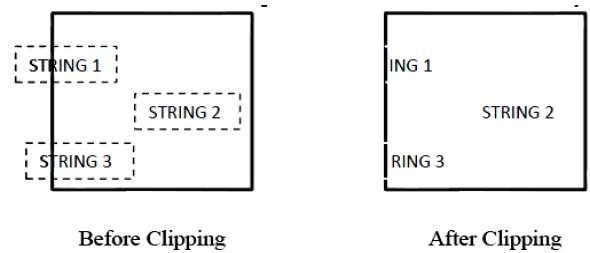This clipping method is based on characters rather than entire string. In this method if the string is entirely inside the clipping window, then we keep it. If it is partially outside the window, then −

• You reject only the portion of the string being outside
• If the character is on the boundary of the clipping window, then we discard that entire character and keep the rest string.

The following figure shows text clipping −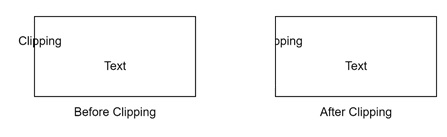This clipping method is based on characters rather than the entire string. In this method if the string is entirely inside the clipping window, then we keep it. If it is partially outside the window, then

• You reject only the portion of string being outside.
• If the character is on the boundary of the clipping window, then we discard only that portion of character that is outside of the clipping window.
Posted in Computer graphics MCA

## Polygon Clipping (Sutherland Hodgman Algorithm)

A polygon can also be clipped by specifying the clipping window. Sutherland Hodgeman polygon clipping algorithm is used for polygon clipping. In this algorithm, all the vertices of the polygon are clipped against each edge of the clipping window.

First the polygon is clipped against the left edge of the polygon window to get new vertices of the polygon. These new vertices are used to clip the polygon against right edge, top edge, bottom edge, of the clipping window as shown in the following figure.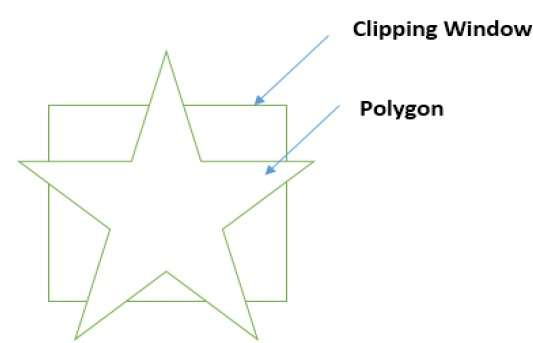While processing an edge of a polygon with clipping window, an intersection point is found if edge is not completely inside clipping window and the a partial edge from the intersection point to the outside edge is clipped. The following figures show left, right, top and bottom edge clippings −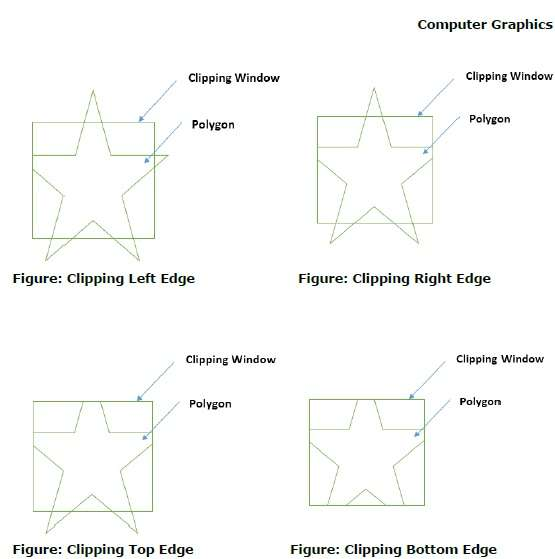## Text Clipping

Various techniques are used to provide text clipping in a computer graphics. It depends on the methods used to generate characters and the requirements of a particular application. There are three methods for text clipping which are listed below −

• All or none string clipping
• All or none character clipping
• Text clipping

The following figure shows all or none string clipping −In all or none string clipping method, either we keep the entire string or we reject entire string based on the clipping window. As shown in the above figure, STRING2 is entirely inside the clipping window so we keep it and STRING1 being only partially inside the window, we reject.

The following figure shows all or none character clipping −This clipping method is based on characters rather than entire string. In this method if the string is entirely inside the clipping window, then we keep it. If it is partially outside the window, then −

• You reject only the portion of the string being outside
• If the character is on the boundary of the clipping window, then we discard that entire character and keep the rest string.

The following figure shows text clipping −This clipping method is based on characters rather than the entire string. In this method if the string is entirely inside the clipping window, then we keep it. If it is partially outside the window, then

• You reject only the portion of string being outside.
• If the character is on the boundary of the clipping window, then we discard only that portion of character that is outside of the clipping window.

## Bitmap Graphics

A bitmap is a collection of pixels that describes an image. It is a type of computer graphics that the computer uses to store and display pictures. In this type of graphics, images are stored bit by bit and hence it is named Bit-map graphics. For better understanding let us consider the following example where we draw a smiley face using bit-map graphics.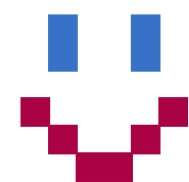Now we will see how this smiley face is stored bit by bit in computer graphics.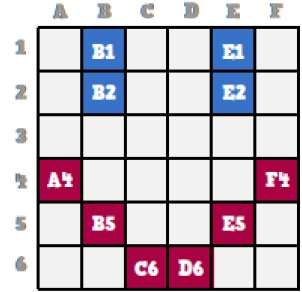By observing the original smiley face closely, we can see that there are two blue lines which are represented as B1, B2 and E1, E2 in the above figure.

In the same way, the smiley is represented using the combination bits of A4, B5, C6, D6, E5, and F4 respectively.

The main disadvantages of bitmap graphics are −

• We cannot resize the bitmap image. If you try to resize, the pixels get blurred.
• Colored bitmaps can be very large.
Posted in Computer graphics MCA

## Line Clipping

The concept of line clipping is same as point clipping. In line clipping, we will cut the portion of line which is outside of window and keep only the portion that is inside the window.

## Cohen-Sutherland Line Clippings

This algorithm uses the clipping window as shown in the following figure. The minimum coordinate for the clipping region is $(XW_{min,} YW_{min})$ and the maximum coordinate for the clipping region is $(XW_{max,} YW_{max})$.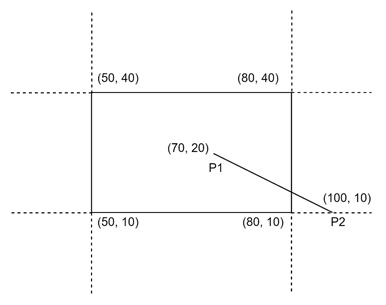We will use 4-bits to divide the entire region. These 4 bits represent the Top, Bottom, Right, and Left of the region as shown in the following figure. Here, the TOP and LEFT bit is set to 1 because it is the TOP-LEFT corner.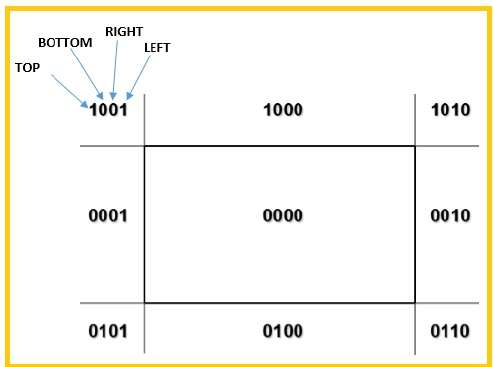There are 3 possibilities for the line −

• Line can be completely inside the window (This line should be accepted).
• Line can be completely outside of the window (This line will be completely removed from the region).
• Line can be partially inside the window (We will find intersection point and draw only that portion of line that is inside region).

### Algorithm

Step 1 − Assign a region code for each endpoints.

Step 2 − If both endpoints have a region code 0000 then accept this line.

Step 3 − Else, perform the logical ANDoperation for both region codes.

Step 3.1 − If the result is not 0000, then reject the line.

Step 3.2 − Else you need clipping.

Step 3.2.1 − Choose an endpoint of the line that is outside the window.

Step 3.2.2 − Find the intersection point at the window boundary (base on region code).

Step 3.2.3 − Replace endpoint with the intersection point and update the region code.

Step 3.2.4 − Repeat step 2 until we find a clipped line either trivially accepted or trivially rejected.

Step 4 − Repeat step 1 for other lines.

## Cyrus-Beck Line Clipping Algorithm

This algorithm is more efficient than Cohen-Sutherland algorithm. It employs parametric line representation and simple dot products.Parametric equation of line is −

P0P1:P(t) = P0 + t(P1 - P0)


Let Ni be the outward normal edge Ei. Now pick any arbitrary point PEi on edge Ei then the dot product Ni.[P(t) – PEi] determines whether the point P(t) is “inside the clip edge” or “outside” the clip edge or “on” the clip edge.

The point P(t) is inside if Ni.[P(t) – PEi] < 0

The point P(t) is outside if Ni.[P(t) – PEi] > 0

The point P(t) is on the edge if Ni.[P(t) – PEi] = 0 (Intersection point)

Ni.[P(t) – PEi] = 0

Ni.[ P0 + t(P1 – P0) – PEi] = 0 (Replacing P(t) with P0 + t(P1 – P0))

Ni.[P0 – PEi] + Ni.t[P1 – P0] = 0

Ni.[P0 – PEi] + Ni∙tD = 0 (substituting D for [P1 – P0])

Ni.[P0 – PEi] = – Ni∙tD

The equation for t becomes,

$$t = \tfrac{N_{i}.[P_{o} – P_{Ei}]}{{- N_{i}.D}}$$

It is valid for the following conditions −

• Ni ≠ 0 (error cannot happen)
• D ≠ 0 (P1 ≠ P0)
• Ni∙D ≠ 0 (P0P1 not parallel to Ei)
Posted in Computer graphics MCA

### Point Clipping Algorithm in Computer Graphics

Clipping: In computer graphics our screen act as a 2-D coordinate system. it is not necessary that each and every point can be viewed on our viewing pane(i.e. our computer screen). We can view points, which lie in particular range (0,0) and (Xmax, Ymax). So, clipping is a procedure that identifies those portions of a picture that are either inside or outside of our viewing pane.
In case of point clipping, we only show/print points on our window which are in range of our viewing pane, others points which are outside the range are discarded.
Example

Input :Output :Point Clipping Algorithm:

1. Get the minimum and maximum coordinates of both viewing pane.
2. Get the coordinates for a point.
3. Check whether given input lies between minimum and maximum coordinate of viewing pane.
4. If yes display the point which lies inside the region otherwise discard it.
5.  // C program for point clipping Algorithm  #include  //#include    // Function for point clipping  void pointClip(int XY[], int n, int Xmin, int Ymin,                                     int Xmax, int Ymax)  {      /*************** Code for graphics view      // initialize graphics mode      detectgraph(&gm,&gr);      initgraph(&gm,&gr,"d:\\tc\\BGI");      for (int i=0; i= Xmin) && (XY[i] <= Xmax))      {              if ( (XY[i] >= Ymin) && (XY[i] <= Ymax))          putpixel(XY[i],XY[i],3);      }      }      **********************/     /**** Arithmetic view ****/     printf ("Point inside the viewing pane:\n");      for (int i=0; i= Xmin) && (XY[i] <= Xmax))          {              if ((XY[i] >= Ymin) && (XY[i] <= Ymax))                  printf ("[%d, %d] ", XY[i], XY[i]);          }      }        // print point coordinate outside viewing pane      printf ("\nPoint outside the viewing pane:\n");      for (int i=0; i Xmax))              printf ("[%d, %d] ", XY[i], XY[i]);          if ((XY[i] < Ymin) || (XY[i] > Ymax))              printf ("[%d, %d] ", XY[i], XY[i]);      }  }    // Driver code  int main()  {      int XY = {{10,10}, {-10,10}, {400,100},                      {100,400}, {400,400}, {100,40}};        // getmaxx() & getmaxy() will return Xmax, Ymax      // value if graphics.h is included      int Xmin = 0;      int Xmax = 350;      int Ymin = 0;      int Ymax = 350;      pointClip(XY, 6,  Xmin, Ymin, Xmax, Ymax);      return 0;  }

Output:

Point inside the viewing pane:
[10, 10] [100, 40]

Point outside the viewing pane:
[-10, 10] [400, 100] [100, 400] [400, 400] [400, 400]
Posted in Computer graphics MCA

### Viewing and Clipping

The primary use of clipping in computer graphics is to remove objects, lines, or line segments that are outside the viewing pane. The viewing transformation is insensitive to the position of points relative to the viewing volume − especially those points behind the viewer − and it is necessary to remove these points before generating the view.

## Point Clipping

Clipping a point from a given window is very easy. Consider the following figure, where the rectangle indicates the window. Point clipping tells us whether the given point (X, Y) is within the given window or not; and decides whether we will use the minimum and maximum coordinates of the window.

The X-coordinate of the given point is inside the window, if X lies in between Wx1 ≤ X ≤ Wx2. Same way, Y coordinate of the given point is inside the window, if Y lies in between Wy1 ≤ Y ≤ Wy2.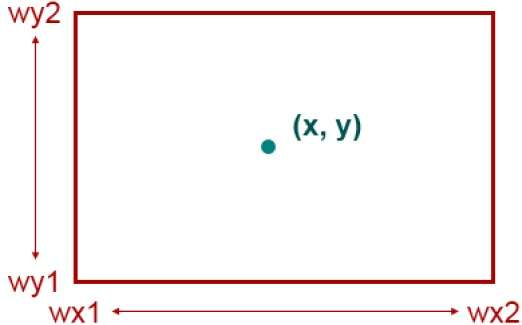## Line Clipping

The concept of line clipping is same as point clipping. In line clipping, we will cut the portion of line which is outside of window and keep only the portion that is inside the window.

## Cohen-Sutherland Line Clippings

This algorithm uses the clipping window as shown in the following figure. The minimum coordinate for the clipping region is $(XW_{min,} YW_{min})$ and the maximum coordinate for the clipping region is $(XW_{max,} YW_{max})$.We will use 4-bits to divide the entire region. These 4 bits represent the Top, Bottom, Right, and Left of the region as shown in the following figure. Here, the TOP and LEFT bit is set to 1 because it is the TOP-LEFT corner.There are 3 possibilities for the line −

• Line can be completely inside the window (This line should be accepted).
• Line can be completely outside of the window (This line will be completely removed from the region).
• Line can be partially inside the window (We will find intersection point and draw only that portion of line that is inside region).

### Algorithm

Step 1 − Assign a region code for each endpoints.

Step 2 − If both endpoints have a region code 0000 then accept this line.

Step 3 − Else, perform the logical ANDoperation for both region codes.

Step 3.1 − If the result is not 0000, then reject the line.

Step 3.2 − Else you need clipping.

Step 3.2.1 − Choose an endpoint of the line that is outside the window.

Step 3.2.2 − Find the intersection point at the window boundary (base on region code).

Step 3.2.3 − Replace endpoint with the intersection point and update the region code.

Step 3.2.4 − Repeat step 2 until we find a clipped line either trivially accepted or trivially rejected.

Step 4 − Repeat step 1 for other lines.

## Cyrus-Beck Line Clipping Algorithm

This algorithm is more efficient than Cohen-Sutherland algorithm. It employs parametric line representation and simple dot products.Parametric equation of line is −

P0P1:P(t) = P0 + t(P1 - P0)


Let Ni be the outward normal edge Ei. Now pick any arbitrary point PEi on edge Ei then the dot product Ni.[P(t) – PEi] determines whether the point P(t) is “inside the clip edge” or “outside” the clip edge or “on” the clip edge.

The point P(t) is inside if Ni.[P(t) – PEi] < 0

The point P(t) is outside if Ni.[P(t) – PEi] > 0

The point P(t) is on the edge if Ni.[P(t) – PEi] = 0 (Intersection point)

Ni.[P(t) – PEi] = 0

Ni.[ P0 + t(P1 – P0) – PEi] = 0 (Replacing P(t) with P0 + t(P1 – P0))

Ni.[P0 – PEi] + Ni.t[P1 – P0] = 0

Ni.[P0 – PEi] + Ni∙tD = 0 (substituting D for [P1 – P0])

Ni.[P0 – PEi] = – Ni∙tD

The equation for t becomes,

$$t = \tfrac{N_{i}.[P_{o} – P_{Ei}]}{{- N_{i}.D}}$$

It is valid for the following conditions −

• Ni ≠ 0 (error cannot happen)
• D ≠ 0 (P1 ≠ P0)
• Ni∙D ≠ 0 (P0P1 not parallel to Ei)

## Polygon Clipping (Sutherland Hodgman Algorithm)

A polygon can also be clipped by specifying the clipping window. Sutherland Hodgeman polygon clipping algorithm is used for polygon clipping. In this algorithm, all the vertices of the polygon are clipped against each edge of the clipping window.

First the polygon is clipped against the left edge of the polygon window to get new vertices of the polygon. These new vertices are used to clip the polygon against right edge, top edge, bottom edge, of the clipping window as shown in the following figure.While processing an edge of a polygon with clipping window, an intersection point is found if edge is not completely inside clipping window and the a partial edge from the intersection point to the outside edge is clipped. The following figures show left, right, top and bottom edge clippings −## Text Clipping

Various techniques are used to provide text clipping in a computer graphics. It depends on the methods used to generate characters and the requirements of a particular application. There are three methods for text clipping which are listed below −

• All or none string clipping
• All or none character clipping
• Text clipping

The following figure shows all or none string clipping −In all or none string clipping method, either we keep the entire string or we reject entire string based on the clipping window. As shown in the above figure, STRING2 is entirely inside the clipping window so we keep it and STRING1 being only partially inside the window, we reject.

The following figure shows all or none character clipping −This clipping method is based on characters rather than entire string. In this method if the string is entirely inside the clipping window, then we keep it. If it is partially outside the window, then −

• You reject only the portion of the string being outside
• If the character is on the boundary of the clipping window, then we discard that entire character and keep the rest string.

The following figure shows text clipping −This clipping method is based on characters rather than the entire string. In this method if the string is entirely inside the clipping window, then we keep it. If it is partially outside the window, then

• You reject only the portion of string being outside.
• If the character is on the boundary of the clipping window, then we discard only that portion of character that is outside of the clipping window.

## Bitmap Graphics

A bitmap is a collection of pixels that describes an image. It is a type of computer graphics that the computer uses to store and display pictures. In this type of graphics, images are stored bit by bit and hence it is named Bit-map graphics. For better understanding let us consider the following example where we draw a smiley face using bit-map graphics.Now we will see how this smiley face is stored bit by bit in computer graphics.By observing the original smiley face closely, we can see that there are two blue lines which are represented as B1, B2 and E1, E2 in the above figure.

In the same way, the smiley is represented using the combination bits of A4, B5, C6, D6, E5, and F4 respectively.

The main disadvantages of bitmap graphics are −

• We cannot resize the bitmap image. If you try to resize, the pixels get blurred.
• Colored bitmaps can be very large.
Posted in Computer graphics MCA

### Window to view port coordinate transformation